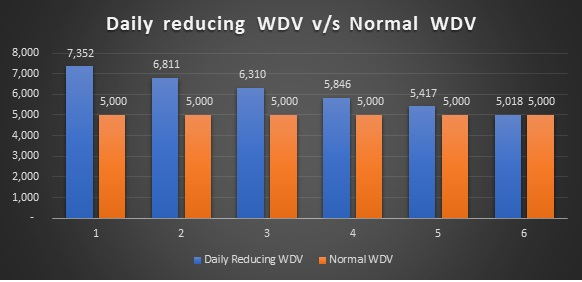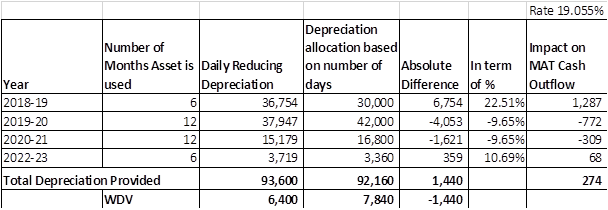prpri Application of Time Value of Money concept to WDV Depreciation Calculation Application of Time Value of Money concept to WDV Depreciation Calculation

# Application of Time Value of Money concept to WDV Depreciation Calculation

Depreciation as per written down value method (WDV) has been under the spotlight after the introduction of the Companies Act, 2013. Schedule II of the act mandates to provide depreciation as per useful life of assets using either straight line method or written down value method. This article is on Application of Time Value of Money Concept to the calculation of Depreciation under WDV method. Let’s start with example;

Example 1

Company A has procured a plant and machinery on 1 September 2018 and put into use on 1 October 2018. Cost of machinery is Rs.50 lacs and useful life is 8 years. Company follows financial year. As per normal practice, when asset is acquired during the year, first year depreciation is calculated based number of days asset is used during the year. In the given case, depreciation for first year will be Rs. 7,78,611 (Rs.50,00,000*31.23%*182/365).

Technically, this is under booking of depreciation. Depreciation for the first year will be Rs.8,53,749. This amount has been derived using time value of money concept, present value formula and charts. In simple term, it is daily reducing WDV depreciation or Compounding WDV Depreciation.

Why this happened so?

In normal WDV Depreciation calculation, depreciation is calculated first annually then it is allocated based on number of days asset used during the year. This allocation becomes straight lined. Let’s take one more example,

Example 2

A computer costing Rs.1 lac has been procured on 1st October 2018. Now, say 60% is rate of WDV. Estimate Residual value Rs.6,400. Useful life is 3 years.

Depreciation = Rs.1,00,000* 60%* 6/12 = Rs.30,000 which makes per month allocation Rs.5,000

During the year, allocation of depreciation becomes straight lined rather than reducing balance.

Under Daily reducing WDV depreciation, depreciation during the initial month is high. Below chart explains the same;Issues under Normal WDV Depreciation Calculation

• At the end of the year, WDV will not equate to residual value.
• Where there is quarterly reporting, WDV are carried forward incorrect.

Impact of using Daily reducing WDV Depreciation/Compounding WDV Depreciation

• As observed above, this method accounts higher depreciation during initial years, this can materially reduce MAT tax liability of company.
• Impact on deferred tax assets and liability.
• Impact on written down value of assets.
• At the end of useful life, WDV matches to residual value of assets.

Year wise Impact analysis explained with 2nd example in below table.Depreciation under daily reducing method is high in initial years which has an impact of saving MAT Tax liability. Further, at the end of the useful life, under normal depreciation, WDV does not equate to residual value.

Myself CA Vishal Mulchandani, I came across to this daily reducing method of depreciation while developing perpetual depreciation calculator. In my opinion, application of time value of money concept to the WDV Depreciation calculation makes more logical and accurate.

Feedback, suggestions are welcome.

CA Vishal Mulchandani – vishalg.mulchandani@gmail.com

#### Author Bio

Qualification: CA in Job / Business
Company: Black Horse Excellence
Member Since: 26 Feb 2019 | Total Posts: 7

July 2021
M T W T F S S
1234
567891011
12131415161718
19202122232425
262728293031